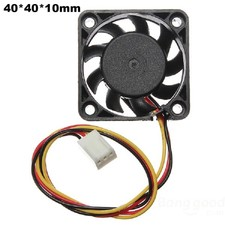User Name Remember Me? Password

 Advanced Thermodynamics Advanced Thermodynamics Physics Help ForumSep 26th 2018, 02:06 AM #1 Junior Member   Join Date: Sep 2018 Posts: 2 Heat loss within a control volume Hello, I have the following problem: I got an electrical component within a chamber and the chamber has an opening, where air can be supplied from and an outlet. Attached you can see an illustration of the problem.The electrical component has a heat loss of 150 W The temperature at the Inlet is known, to be lets say $\displaystyle T_1 = 30°C / 303 K$ The volume of the chamber is $\displaystyle V =0.014 m³$ The surface area of the eletctrical component is $\displaystyle A = 0.37 m²$ What I want to know is:What is the temperature inside the chamber? Also, what is the temperature at the outlet? Can it be assumed, that it has the same temperature as the chamber? At the end I would like to determine, which mass flow I need, to keep the temperature in the chamber below 70 °C The first thing I attempted by calculating the mass flow with the use of $\displaystyle Q = \alpha A (T_{surface} - T_1 )$ where I can rewrite the equation to the mass flow by rewriting the heat transfer coefficient alpha with the Nusselt correlation. Furthermore, in order to solve for the mass flow rate I assumed the surface temperature to be 70°C as this is my maximum. Is this the correct way to solve it or do I have to consider something else as well? I also had an attempt to set up the energy balance by $\displaystyle E_{in} = E_{out}$ $\displaystyle W_{in, electrical} + m h_1 = \alpha A (T_2 - T_1) + m h_2$ thus, $\displaystyle T_2 = W_{in, electrical} / (\alpha A + m c_p) + T_1$ which I can calculate for different mass flow rates. I assumed to be the surface temperature equal to $\displaystyle T_2$ I think, that there is a mistake in this equation but do not know exactly how to set it up differently. *The Q, W and m are all rate thus they should be seen with a point above them (don´t know how to insert it) I have also made the assumption to keep it simple at first to just consider convection - can that assumption be made? I hope it is clear. Thanks for the help in advance!Sep 27th 2018, 03:55 AM #2 Member   Join Date: Sep 2014 Location: Brasília, DF - Brazil Posts: 32 let's assume isolated walls e a VC surrounding all the chamber: For steady state and using the lumped formulation of 1st law of thermodynamics: $\displaystyle 0=\dot{Q}_{gen}+\dot{m}_{in}h_{in}-\dot{m}_{out}h_{out}$ For $\displaystyle \dot{m}_{in}=\dot{m}_{out}$ $\displaystyle \dot{Q}_{gen}=\dot{m}\Delta h$ $\displaystyle \dot{Q}_{gen}=\dot{m}\Delta h$ Assuming constant pressure: $\displaystyle \dot{Q}_{gen}=\dot{m}c_p\Delta T$ and now you can calculate $\displaystyle T_{out}$ In this problem, in general, its temperature profile is in the form T (t, x, y). I'm not sure how deep is this problem, but it's possible to assume $\displaystyle T_{chamber}=T_{out}$ You could calculate Prandt and Reynolds to find Nusselt and use external flow approach but i don't how deep is your problem __________________ Work on: General thermal systems Cryogenics Micro-drop fluid mechanics Last edited by mscfd; Sep 27th 2018 at 09:12 AM.Sep 27th 2018, 06:40 AM #3 Senior Member   Join Date: Apr 2017 Posts: 513 We have heat output 150W .... input air temp 30C ... what will be the air output temp at different flow rates??? specific heat of air is close to 1 J/gmC so for an output temp of 50C ... 150/20 = 7.5 liters /sec ............ output temp of 40C ......... 15 liters /sec These are quiet modest air flow rates ........... you haven't given the diameters of the inlet and outlet holes ... I would just buy a computer fan to suit the hole size ... measure the outlet temp .. the flow will be so fast it won't be above 50C and will keep your component plenty cool ..this one costs \$1 inc delivery , eBay ... will suit a hole 35mm diaOct 8th 2018, 02:45 AM #4 Junior Member   Join Date: Sep 2018 Posts: 2 Thanks for the answers!Tags control, heat, loss, volumeThread ToolsShow Printable VersionEmail this Page Display ModesLinear ModeSwitch to Hybrid ModeSwitch to Threaded ModeSimilar Physics Forum Discussions Thread Thread Starter Forum Replies Last Post DallasSE Advanced Thermodynamics 8 Nov 13th 2017 06:26 AM SpiltShift22 Advanced Thermodynamics 2 Apr 15th 2016 05:03 AM Hero Energy and Work 27 Nov 9th 2015 02:49 PM SwireWS Advanced Thermodynamics 2 Oct 31st 2015 04:49 AM drone007 Thermodynamics and Fluid Mechanics 4 May 30th 2014 02:35 PM